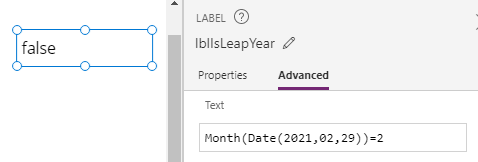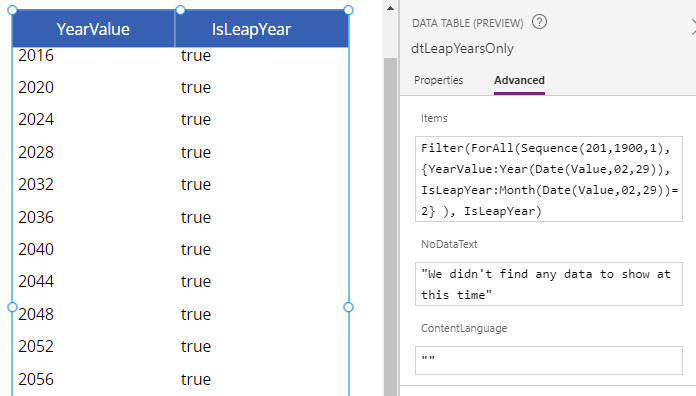# Dates - How to check if a year is a leap year

Working with dates, it can sometimes be necessary to work out whether a given year is a leap year. This post describes a technique we can use to carry out this task.

With Power Apps, there is no built-in function to return whether a given year is a leap year. However, we can apply a relatively simple technique to determine whether a year is a leap, which we'll cover in this post.

## How to determine if a year is a leap year

A technique we can use to determine whether a year is a leap year is to attempt to call the Date function to define a date value for the target year with a day and month of 29th February.

The interesting behaviour is this. If 29th February doesn't exist for the target year, the Date function returns the 1st March for the target year instead (as highlighted in the screenshot beneath).Because of this behaviour, we can then call the Month function against this return value. If the month value equals 2, this verifies that the input year is a leap year. If the month value equals 3, the year is not a leap year.

As a demonstration, the formula beneath returns false because 2021 is not a leap year.

`Month(Date(2021,2,29))=2`## Creating a list of years with the leap year status

To provide a further example of how to apply this technique, we can create a list of years and include a field that indicates whether the year is a leap year.

The formula beneath creates a sequence of years starting with the year 1900. For each year in this sequence, we use our technique to add a field that indicates whether we can correctly declare a date value with the day and month of 29th February.

`ForAll(Sequence(200,1900,1),       {         YearValue: Year(Date(Value,2,29)),          IsLeapYear: Month(Date(Value,2,29))=2      }) `
The screenshot beneath illustrates the formula as applied to a data table control.## Create a list of leap years

To create a list of leap years, we can filter our example from above to return only those records where the IsLeapYear field equals true.

`Filter(   ForAll(Sequence(200,1900,1),          {            YearValue: Year(Date(Value,2,29)),             IsLeapYear: Month(Date(Value,2,29))=2               }    ),    IsLeapYear) `
The screenshot beneath illustrates the output.## Conclusion

To determine if a year is a leap year, we can attempt to declare a date with the day and month of 29th February. If this succeeds, the year is a leap year.